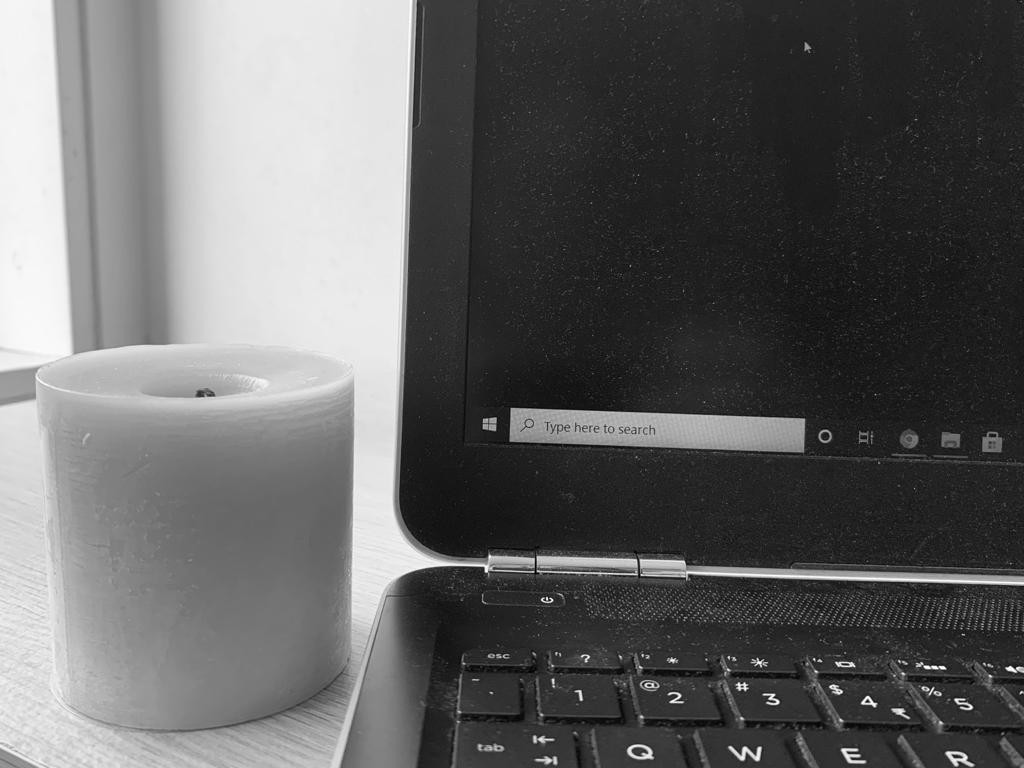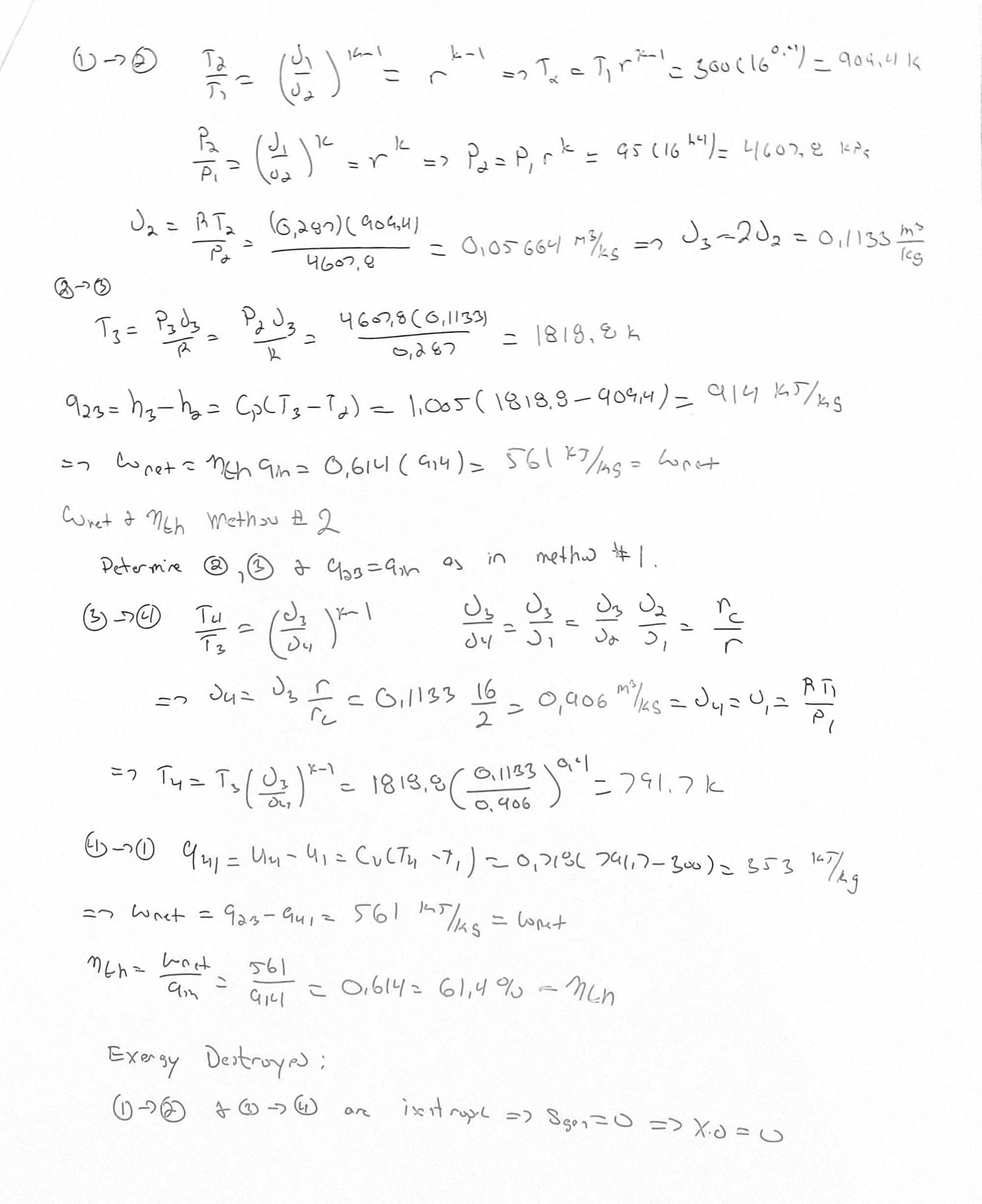# 用Numpy，Open-CV增强灰度图像1. 将必要的软件包导入你的环境。为了易于显示图像，我们使用Jupyter Notebook。
``````import cv2
import numpy as np
import matplotlib.pyplot as plt

1. 删除阴影时，有两件事要注意。由于图像是灰度图像，如果图像背景较浅且对象较暗，则必须先执行最大值滤波，然后再执行最小值滤波。如果图像背景较暗且物体较亮，我们可以先执行最小值滤波，然后再进行最大值滤波。

1. 最大值滤波：让我们假设我们有一个特定大小的图像 I 。我们编写的算法应逐个遍历 I 的像素，并且对于每个像素（x，y），它必须找到该像素周围的邻域（大小为N x N的窗口）中的最大灰度值，并将该最大灰度值写入A中相应的像素位置（x，y）。所得图像 A 称为输入图像 I 的最大值滤波图像。

• max_filtering()函数接受输入图像和窗口大小N。

• 它最初在输入数组周围创建一个“wall”（带有-1的填充），当我们遍历边缘像素时会有所帮助。

• 然后，我们创建一个“ temp”变量，将计算出的最大值复制到该变量中。

• 然后，我们遍历数组，并围绕当前像素大小N x N创建一个窗口。

• 然后，我们使用“ amax()”函数在该窗口中计算最大值，并将该值写入temp数组。

• 我们将该临时数组复制到主数组A中，并将其作为输出返回。

• A是输入I的最大值滤波图像。

``````def max_filtering(N, I_temp):
wall = np.full((I_temp.shape+(N//2)*2, I_temp.shape+(N//2)*2), -1)
wall[(N//2):wall.shape-(N//2), (N//2):wall.shape-(N//2)] = I_temp.copy()
temp = np.full((I_temp.shape+(N//2)*2, I_temp.shape+(N//2)*2), -1)
for y in range(0,wall.shape):
for x in range(0,wall.shape):
if wall[y,x]!=-1:
window = wall[y-(N//2):y+(N//2)+1,x-(N//2):x+(N//2)+1]
num = np.amax(window)
temp[y,x] = num
A = temp[(N//2):wall.shape-(N//2), (N//2):wall.shape-(N//2)].copy()
return A

1. 最小值滤波：此算法与最大值滤波完全相同，但是我们不去找邻近的最大灰度值，而是找到了该像素周围N x N邻近的最小值，并将该最小灰度值写入B中的（x，y）。所得的图像 B 称为图像 I 的经过最小值滤波的图像。

``````def min_filtering(N, A):
wall_min = np.full((A.shape+(N//2)*2, A.shape+(N//2)*2), 300)
wall_min[(N//2):wall_min.shape-(N//2), (N//2):wall_min.shape-(N//2)] = A.copy()
temp_min = np.full((A.shape+(N//2)*2, A.shape+(N//2)*2), 300)
for y in range(0,wall_min.shape):
for x in range(0,wall_min.shape):
if wall_min[y,x]!=300:
window_min = wall_min[y-(N//2):y+(N//2)+1,x-(N//2):x+(N//2)+1]
num_min = np.amin(window_min)
temp_min[y,x] = num_min
B = temp_min[(N//2):wall_min.shape-(N//2), (N//2):wall_min.shape-(N//2)].copy()
return B

1. 因此，如果图像的背景较浅，我们要先执行最大值滤波，这将为我们提供增强的背景，并将该最大值滤波后的图像传递给最小值滤波函数，该函数将负责实际的内容增强。

2. 因此，执行最小-最大值滤波后，我们获得的值不在0-255的范围内。因此，我们必须归一化使用背景减法获得的最终阵列，该方法是用原始图像减去最小最大值滤波后的图像，以获得去除了阴影的最终图像。

``````#B is the filtered image and I is the original image
def background_subtraction(I, B):
O = I - B
norm_img = cv2.normalize(O, None, 0,255, norm_type=cv2.NORM_MINMAX)
return norm_img

1. 变量N（用于过滤的窗口大小）将根据图像中粒子或内容的大小进行更改。对于测试图像，选择大小N = 20。增强后的最终输出图像如下所示：sklearn机器学习中文官方文档： sklearn123.com/